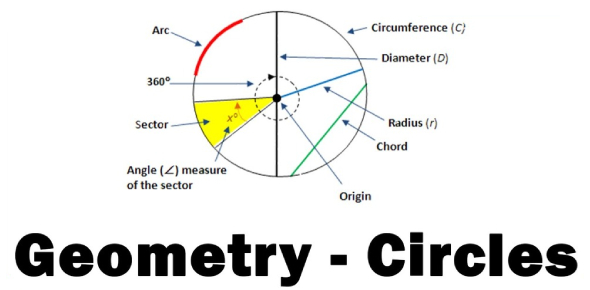Geometry And The Circle! Math Trivia Questions Quiz

30 Questions | Total Attempts: 73Settings.

• 1.
Use the equation box to match the circle with the equation.  Write the alphabet on the line provided.
• 2.
Use the equation box to match the circle with the equation.  Write the alphabet on the line provided.
• 3.
Use the equation box to match the circle with the equation.  Write the alphabet on the line provided.
• 4.
Use the equation box to match the circle with the equation.  Write the alphabet on the line provided.
• 5.
Use the equation box to match the circle with the equation.  Write the alphabet on the line provided.
• 6.
Use the equation box to match the circle with the equation.  Write the alphabet on the line provided.
• 7.
Use the equation box to match the circle with the equation.  Write the alphabet on the line provided.
• 8.
Use the equation box to match the circle with the equation.  Write the alphabet on the line provided.
• 9.
Use the equation box to match the circle with the equation.  Write the alphabet on the line provided.
• 10.
Use the equation box to match the circle with the equation.  Write the alphabet on the line provided.
• 11.
Find the measure of the arc or central angle indicated. Assume that lines which appear to be diameters are actual diameters.
• 12.
Find the measure of the arc or central angle indicated. Assume that lines which appear to be diameters are actual diameters.
• 13.
Find the measure of the arc or central angle indicated. Assume that lines which appear to be diameters are actual diameters.
• 14.
Find the measure of the arc or central angle indicated. Assume that lines which appear to be diameters are actual diameters.
• 15.
Find the area of each. Use your calculator's value of [pi]. Round your answer to the nearest tenth.
• 16.